# Inverse Tangent Function (Arctangent)

Each of the trigonometric functions sine, cosine, tangent, secant, cosecant and cotangent has an inverse (with a restricted domain). The inverse is used to obtain the measure of an angle using the ratios from basic right triangle trigonometry. The inverse of tangent is denoted as Arctangent or on a calculator it will appear as atan or tan-1. Note: this does NOT mean tangent raised to the negative one power.

Let's look at an example of how to use the inverse tangent function to find the measure of an angle in a right triangle. (triangle not drawn to scale)To find the measure in degrees of angle A using the tangent inverse, recall that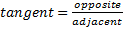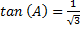Use a scientific calculator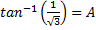*Make sure your calculator is in degree mode

30° = A

Let's look at an application problem.

Squeaky Squirrel is 6 feet from the base of a tree that is 10 feet tall. What is the angle of elevation as Squeaky looks at the top of the tree?

Begin by creating a diagram of the given information.Recall that      tangentθ =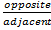Therefore        tangentθ =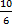Use the inverse of tangent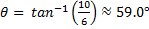rounded to the nearest tenth

If it is understood how to use the basic right triangle trigonometric ratios, then the inverse can be used to find a missing angle measure in any right triangle. If all three sides of the triangle are given then any of the trig ratios can be used and an equivalent angle measure will be derived. Sine, cosine, secant, tangent, cosecant and cotangent are all functions however, the inverses are only a function when given a restricted domain.

 Related Links: Math Trigonometry Graphing a Sine Function Graphing the Tangent Function

To link to this Inverse Tangent Function (Arctangent) page, copy the following code to your site: